Calibrating Electronics Thermal Simulation Models

Internal junction temperature of a package is a key parameter because it’s linked to reliability and functional performance.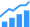‘Rubbish In, Rubbish Out’ is a common and well-accepted fact in the world of thermal simulation—actually any type of simulation, for that matter. Regardless of the technical capabilities of your thermal simulation tool, the accuracy of prediction will always be tightly coupled to the accuracy of the input data.

In terms of electronics thermal simulation, the prediction of the internal junction temperature of a package is often a key parameter of interest. It’s linked to subsequent reliability and functional performance and is itself dependent on all the thermal resistances the heat experiences as it travels from its source at the die, all the way through the package, board, system and final ambient. Calibrating the numerical model to ensure those resistances are accurately defined, especially in the package itself, is a pre-requisite of the simulation process.

The Mentor Graphics T3Ster technology is a non-destructive physical test method that measures the thermal response of a packaged die to a change in its power dissipation. The resulting junction temperature vs. time curve can be mathematically processed, converting it into a ‘structure function’, a graph that describes the thermal resistances and thermal capacitances that the heat flows through (Figure 1). Kind of like a ‘thermal sonar’ approach, insights into construction and material properties can be inferred from this single transient thermal test.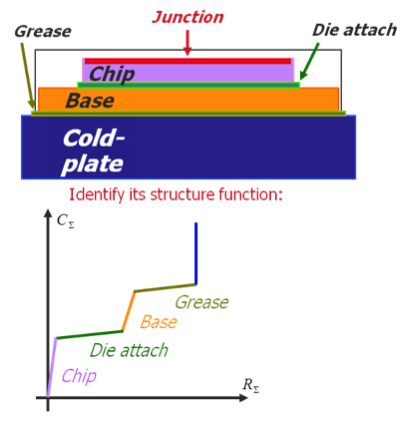Fig. 1: Structure Function Representation of a Packaged IC Stack

A 3D numerical model of the same setup would produce the same (simulated) thermal transient response, and thus the same (numerically derived) structure function. If the two structure functions were seen to deviate, the location of the deviation would be relatable to where the numerical model was wrong (spatially) and by how much (in terms of +/- thermal resistance and capacitance). The numerical model could then be calibrated until it produced the same results that were experimentally measured.

By way of example, this calibration methodology was applied to a simple TO220 package, placed on a copper bar, mounted on a cold plate (Figure 2).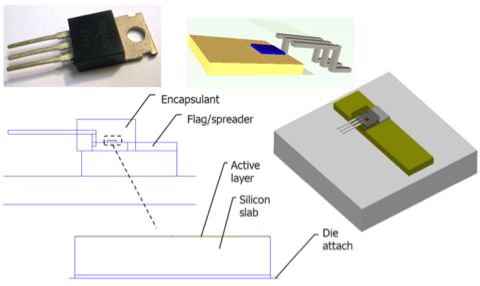Fig. 2: TO220 Simulation Model

The simulation shows how the heat penetrates through the package as it is powered on (Figure 3).

Comparing the resulting structure function with what was experimentally measured shows that the topology of the structure functions match (i.e. the same number of objects are experienced by the heat, in the same order), however the absolute values for thermal resistance and thermal capacitance do not match (Figure 4).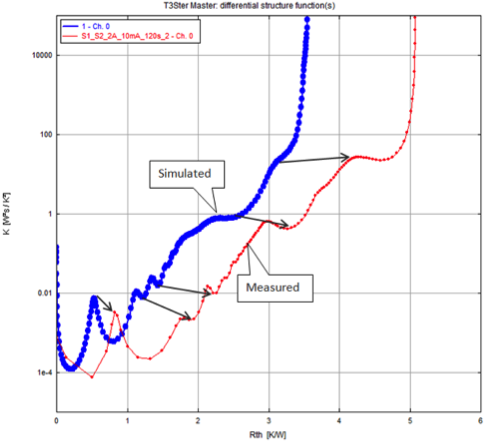Fig. 4: Comparison of Experimental and Simulation Structure Functions

Starting at the die, successive modifications are made to the numerical model, and the simulated structure function re-evaluated, until such time as the simulated and measured structure functions match. The location and the amount of modification are obtained directly from the structure function comparison. The end result is a calibrated numerical model that produces the same thermal response as what was measured. This can be verified by a Zth thermal impedance comparison (Figure 5).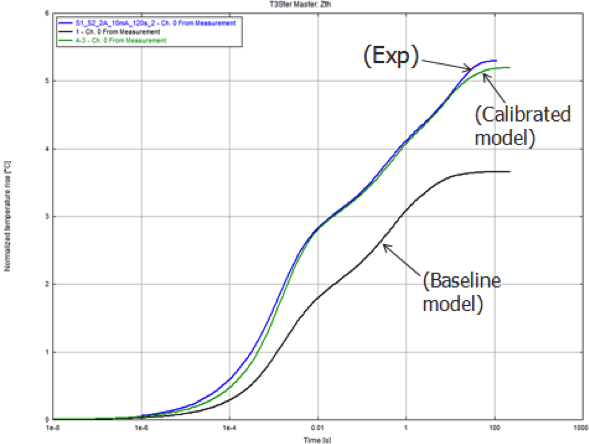Fig. 5: Calibrated Zth Verification

The real beauty of this methodology lies in the amount of insight that the T3Ster measured structure functions provide. A single non-destructive test that can produce a description of all the thermal resistances and thermal capacitances experienced by the heat, a wealth of information, unrivalled by alternative ‘spot testing’ thermocouple type temperature measurement approaches. The calibrated model of the IC package can now go on to be used, with confidence, in the simulation of actual operating conditions, e.g., in terms of power dissipation vs. time and cooling environment in electronics thermal simulation tools such as Mentor’s FloTHERM.